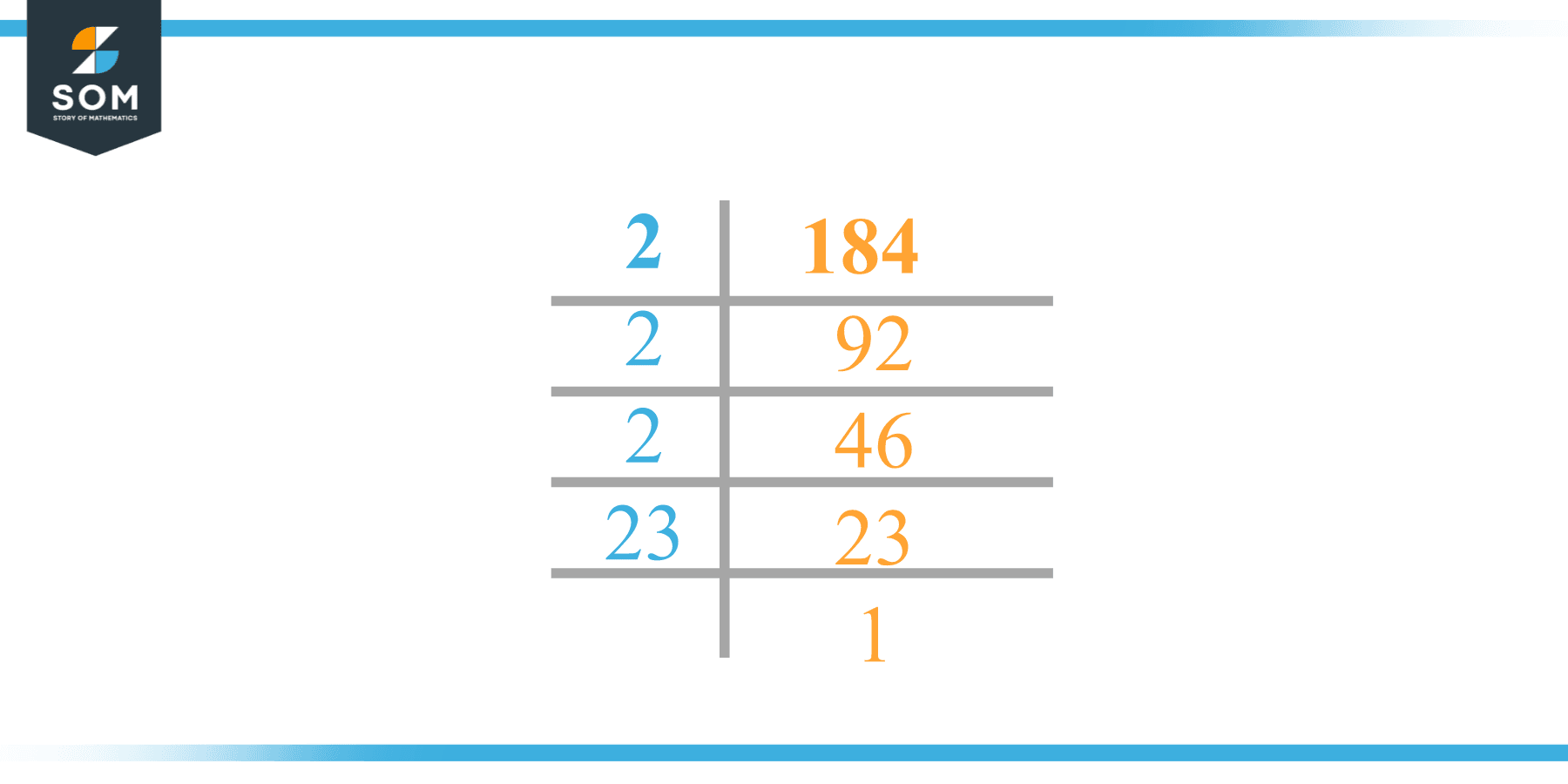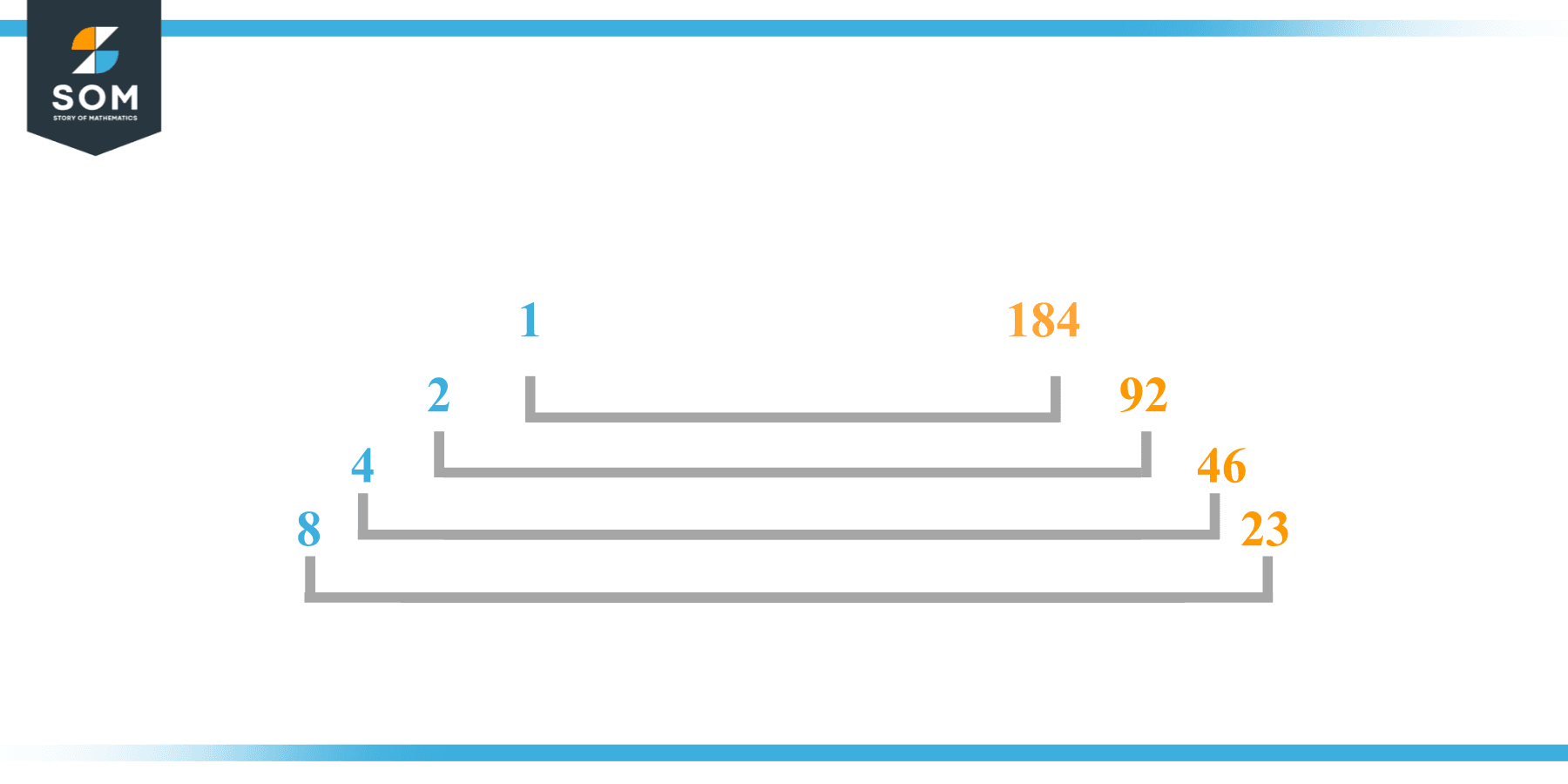# Factors of 184: Prime Factorization, Methods, and Examples

The factors of 184 are the numbers that can be fully divided by 184 without any remainder left. The number 184 is an even composite which concludes that it will have more than two factors.The factors of the given number can be positive as well as negative provided that the given number is achieved upon multiplication of two-factor integers.

### Factors of 184

Here are the factors of number 184.

Factors of 184: 1, 2, 4, 8, 23, 46, 92, 184

### Negative Factors of 184

The negative factors of 184 are similar to its positive factors, just with a negative sign.

Negative Factors of 184: -1, -2, -4, -8, -23, -46, -92, -184

### Prime Factorization of 184

The prime factorization of 184 is the way of expressing its prime factors in the product form.

Prime Factorization: 2 x 2 x 2 x 23

In this article, we will learn about the factors of 184 and how to find them using various techniques such as upside-down division, prime factorization, and factor tree.

## What Are the Factors of 184?

The factors of 184 are 1, 2, 4, 8, 23, 46, 92, and 184. All of these numbers are the factors as they do not leave any remainder when divided by 184.

The factors of 184 are classified as prime numbers and composite numbers. The prime factors of the number 184 can be determined using the technique of prime factorization.

## How To Find the Factors of 184?

You can find the factors of 184 by using the rules of divisibility. The divisibility rule states that any number, when divided by any other natural number, is said to be divisible by the number if the quotient is the whole number and the resulting remainder is zero.

To find the factors of 184, create a list containing the numbers that are exactly divisible by 184 with zero remainders. One important thing to note is that 1 and 184 are the 184’s factors as every natural number has 1 and the number itself as its factor.

1 is also called the universal factor of every number. The factors of 184 are determined as follows:

$\dfrac{184}{1} = 184$

$\dfrac{184}{2} = 92$

$\dfrac{184}{4} = 46$

$\dfrac{184}{8} = 23$

$\dfrac{184}{184} = 1$

Therefore, 1, 2, 4, 8, 23, 46, 92, and 184 are the factors of 184.

### Total Number of Factors of 184

For 184 there are 8 positive factors and 8 negative ones. So in total, there are 16 factors of 184.

To find the total number of factors of the given number, follow the procedure mentioned below:

1. Find the factorization of the given number.
2. Demonstrate the prime factorization of the number in the form of exponent form.
3. Add 1 to each of the exponents of the prime factor.
4. Now, multiply the resulting exponents together. This obtained product is equivalent to the total number of factors of the given number.

By following this procedure the total number of factors of 184 is given as:

Factorization of 184 is 1 x $2^3$ x 21.

The exponent of 1 and 23 is 1. The exponent of 2 is 3.

Adding 1 to each and multiplying them together results in 16.

Therefore, the total number of factors of 184 is 16. 8 are positive and 8 factors are negative.

### Important Notes

Here are some important points that must be considered while finding the factors of any given number:

• The factor of any given number must be a whole number.
• The factors of the number cannot be in the form of decimals or fractions.
• Factors can be positive as well as negative.
• Negative factors are the additive inverse of the positive factors of a given number.
• The factor of a number cannot be greater than that number.
• Every even number has 2 as its prime factor, the smallest prime factor.

## Factors of 184 by Prime FactorizationThe number 184is a composite. Prime factorization is a useful technique for finding the number’s prime factors and expressing the number as the product of its prime factors.

Before finding the factors of 184 using prime factorization, let us find out what prime factors are. Prime factors are the factors of any given number that are only divisible by 1 and themselves.

To start the prime factorization of 184, start dividing by its smallest prime factor. First, determine that the given number is either even or odd. If it is an even number, then 2 will be the smallest prime factor.

Continue splitting the quotient obtained until 1 is received as the quotient. The prime factorization of 184 can be expressed as:

184 = $2^3$ x 23

## Factors of 184 in PairsThe factor pairs are the duplet of numbers that when multiplied together result in the factorized number. Factor pairs can be more than one depending on the total number of factors of the given numbers.

For 184, the factor pairs can be found as:

1 x 184 = 184

2 x 92 = 184

4 x 46 = 184

8 x 23 = 184

The possible factor pairs of 184 are given as (1, 184), (2, 92), (4, 46),  and (8, 23).

All these numbers in pairs, when multiplied, give 184 as the product.

The negative factor pairs of 184 are given as:

-1 x -184 = 184

-2 x -92 = 184

-4 x -46 = 184

-8 x -23 = 184

It is important to note that in negative factor pairs, the minus sign has been multiplied by the minus sign due to which the resulting product is the original positive number. Therefore, -1, -2, -4, -8, -23, -46, -92, and -184 are called negative factors of 184.

The list of all the factors of 184 including positive as well as negative numbers is given below.

Factor list of 184: 1, -1, 2, -2, 4, -4, 8, -8, 23, -23, 46, -46, 92, -92, 184, and -184

## Factors of 184 Solved Examples

To better understand the concept of factors, let’s solve some examples.

### Example 1

How many factors of 184 are there?

### Solution

The total number of Factors of 184 is 8.

Factors of 184 are 1, 2, 4, 8, 23, 46, 92, and 184.

### Example 2

Find the factors of 184 using prime factorization.

### Solution

The prime factorization of 184 is given as:

184 $\div$ 2 = 92

92 $\div$ 2 = 46

46 $\div$ 2 = 23

23 $\div$ 23 = 1

So the prime factorization of 184 can be written as:

$2^3$ x 23 = 184